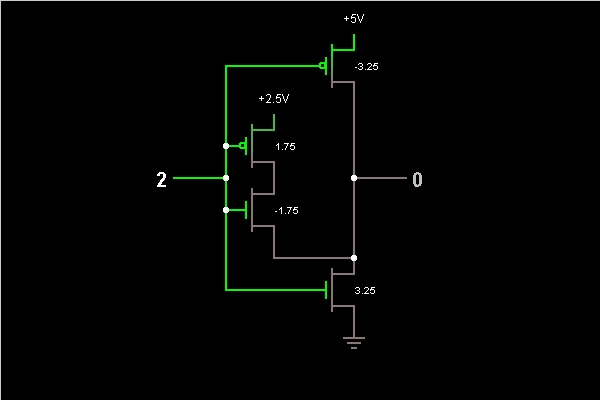# Circuit Simulator - Ternary Logic Inverter

Ternary Logic InverterCircuit Description:
This is an example of a ternary logic gate, implemented using MOSFETs that have the indicated threshold voltages. This gate's input has three states: 0 (ground), 1 (2.5 V), and 2 (5 V). This is an inverter; if the input is X, the output is 2-X.

When the input is 2, the n-MOSFET at lower right pulls the output to ground (the MOSFET switches on whenever the gate voltage is 3.25 V or above). When the input is 0, this MOSFET switches off, and the p-MOSFET on top pulls the output to 5 V (the MOSFET switches on whenever the gate voltage is below 5 V-3.25 V, or 1.75 V). When the input is 1, both the MOSFETs on the right are off, but the two MOSFETs on the left switch on, pulling the output to 2.5 V.

Discussion:
Be the first person to comment on this question !